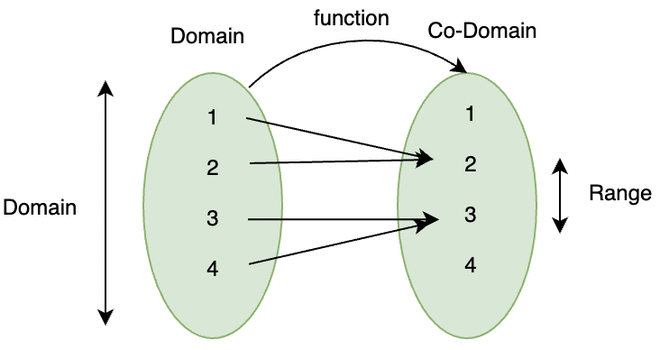Relation and Function

## Objective

To identify a relation and a function.

## Theory

Cartesian Product

If A and B are sets, the cartesian product of A and B is the set A x B = (a, b) : (a ∈ A) and (b ∈ B).

Ordered Pair

An order pair is represented as (input, output) is the relationship between input and output.

Domain

The set of all possible values which qualify as inputs to a function is known as the domain of the function, or it can also be defined as the entire set of values possible for independent variables.

Co-domain

The Co-Domain is the set of all possible outcomes of a given relation or function. Sometimes, the codomain is also equal to the range of the function.

Range

What actually comes out of a function? These ranges are also known as permissible outputs.Relation

• A relation R is the subset of the Cartesian product of X x Y, where X and Y are two non-empty elements. It is derived by stating the relationship between the first element and second element of the ordered pair of X × Y.
• The set of all primary elements of Functionthe ordered pairs is called a domain of R and the set of all second elements of the ordered pairs is called a range of R.

Function

• A relation ‘f’ is said to be a function, if every element of a non-empty set X, has only one image or range to a non-empty set Y.
• A function which has either R or one of its subsets as its range, is called a real valued function. Further, if its domain is also either R or a subset of R, it is called a real function.